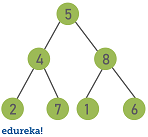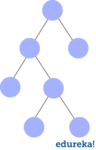# Trees in Java: How to Implement a Binary Tree?13 / 22 Blog from Java Collections

If I had to pick the single most important topic in software development, it would be data structures. One of the most common and easiest ones is a tree – a hierarchical data structure. In this article, let’s explore Trees in Java.

## What is a Binary Tree?

Tree is a non-linear data structure where data objects are generally organized in terms of hierarchical relationship. The structure is non-linear in the sense that, unlike Arrays, Linked Lists, Stack and Queues, data in a tree is not organized linearly. A binary tree is a recursive tree data structure where each node can have 2 children at most.Binary trees have a few interesting properties when they’re perfect:

• Property 1: The number of total nodes on each “level” doubles as you move down the tree.
• Property 2: The number of nodes on the last level is equal to the sum of the number of nodes on all other levels, plus 1

Each data element stored in a tree structure called a node. A Tree node contains the following parts:
1. Data
2. Pointer to left child
3. Pointer to the right child

In Java, we can represent a tree node using class. Below is an example of a tree node with integer data.

```static class Node {
int value;
Node left, right;

Node(int value){
this.value = value;
left = null;
right = null;
} ```

Now that you know what is a binary tree, let’s check out different types of binary trees.

## Types of Binary Trees

### Full Binary Tree

A full binary tree is a binary tree where every node has exactly 0 or 2 children. The example of fully binary tress is:### Perfect Binary Tree

A binary tree is perfect binary Tree if all internal nodes have two children and all leaves are at the same level. The example of perfect binary tress is:### Complete Binary Tree

complete binary tree is a binary tree in which every level, except possibly the last, is completely filled, and all nodes are as far left as possible. An example of a complete binary tree is:Now that you are aware of different types of binary trees, let’s check out how to create a binary tree.

## Binary Tree Implementation

For the implementation, there’s an auxiliary Node class that will store int values and keeps a reference to each child. The first step is to find the place where we want to add a new node in order to keep the tree sorted. We’ll follow these rules starting from the root node:

• if the new node’s value is lower than the current node’s, go to the left child
• if the new node’s value is greater than the current node’s, go to the right child
• when the current node is null, we’ve reached a leaf node, we insert the new node in that position

Now let’s see how we can implement this logic with the help of an example:

```package MyPackage;

public class Tree {
static class Node {
int value;
Node left, right;

Node(int value){
this.value = value;
left = null;
right = null;
}
}

public void insert(Node node, int value) {
if (value < node.value) { if (node.left != null) { insert(node.left, value); } else { System.out.println(" Inserted " + value + " to left of " + node.value); node.left = new Node(value); } } else if (value > node.value) {
if (node.right != null) {
insert(node.right, value);
} else {
System.out.println("  Inserted " + value + " to right of "
+ node.value);
node.right = new Node(value);
}
}
}
public void traverseInOrder(Node node) {
if (node != null) {
traverseInOrder(node.left);
System.out.print(" " + node.value);
traverseInOrder(node.right);
}
}

public static void main(String args[])
{
Tree tree = new Tree();
Node root = new Node(5);
System.out.println("Binary Tree Example");
System.out.println("Building tree with root value " + root.value);
tree.insert(root, 2);
tree.insert(root, 4);
tree.insert(root, 8);
tree.insert(root, 6);
tree.insert(root, 7);
tree.insert(root, 3);
tree.insert(root, 9);
System.out.println("Traversing tree in order");
tree.traverseLevelOrder();

}
}

```

Output:

```Binary Tree Example
Building tree with root value 5
Inserted 2 to left of 5
Inserted 4 to right of 2
Inserted 8 to right of 5
Inserted 6 to left of 8
Inserted 7 to right of 6
Inserted 3 to left of 4
Inserted 9 to right of 8
Traversing tree in order
2 3 4 5 6 7 8 9```

In this example, we have used in-order traversal to traverse the tree. The in-order traversal consists of first visiting the left sub-tree, then the root node, and finally the right sub-tree. There are more ways to traverse a tree. Let’s check them out.

## Tree Traversals

Trees can be traversed in several ways: Let’s use the same tree example that we used before for each case.

### Depth First Search

Depth-first search is a type of traversal where you go as deep as possible down one path before backing up and trying a different one. There are several ways to perform a depth-first search: in-order, pre-order and post-order

We already checked out in-order traversal. Let’s check out pre-order and post-order now.

Pre-Order Traversal

In Pre-order traversal you visit the root node first, then the left subtree, and finally the right subtree. Here’s the code.

```public void traversePreOrder(Node node) {
if (node != null) {
System.out.print(" " + node.value);
traversePreOrder(node.left);
traversePreOrder(node.right);
}
}```

Output:

` 5 2 4 3 8 6 7 9`

Post-Order Traversal

In Post-order traversal you visit left subtree first, then the right subtree, and the root node at the end. Here’s the code.

```public void traversePostOrder(Node node) {
if (node != null) {
traversePostOrder(node.left);
traversePostOrder(node.right);
System.out.print(" " + node.value);
}
}```

Output:

` 3 4 2 7 6 9 8 5`

This type of traversal visits all the nodes of a level before going to the next level. It is like throwing a stone in the center of a pond. The nodes you explore “ripple out” from the starting point. Breadth-First Search is also called level-order and visits all the levels of the tree starting from the root, and from left to right.

## Applications of Binary Tree

Applications of binary trees include:

• Used in many search applications where data is constantly entering/leaving
• As a workflow for compositing digital images for visual effects
• Used in almost every high-bandwidth router for storing router-tables
• Also used in wireless networking and memory allocation
• Used in compression algorithms and many more

This brings us to the end of this ‘Trees in Java’ article.

Data Structures in Java | Stack, Queue, LinkedList, Tree in Data Structures | Edureka

Make sure you practice as much as possible and revert your experience.

Check out the Java Course Online by Edureka, a trusted online learning company with a network of more than 250,000 satisfied learners spread across the globe. We are here to help you with every step on your journey, for becoming a besides this java interview questions, we come up with a curriculum which is designed for students and professionals who want to be a Java Developer.

Got a question for us? Please mention it in the comments section of this ‘Trees in Java’ article and we will get back to you as soon as possible or you can also join our Java Training in Ernakulam.

Upcoming Batches For Java Certification Training Course
Course NameDate
Java Certification Training Course

Class Starts on 25th March,2023

25th March

SAT&SUN (Weekend Batch)
View Details
Java Certification Training Course

Class Starts on 29th April,2023

29th April

SAT&SUN (Weekend Batch)
View DetailsREGISTER FOR FREE WEBINARThank you for registering Join Edureka Meetup community for 100+ Free Webinars each month

### Subscribe to our Newsletter, and get personalized recommendations.#### Trees in Java: How to Implement a Binary Tree?

edureka.co